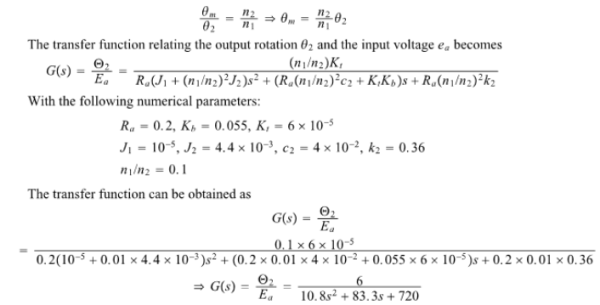In the given simplified dc servomotor the inductance in the armature circuit can be neglected. Also motor damping (c1) and motor spring constant (k1) are both small and negligible. Obtain the transfer function G(s) between the output rotation theta2 and input voltage ea using the shown set of parameters.In the given simplified dc servomotor the inductance in the armature circuit can be neglected. Also motor damping (c1) and motor spring constant (k1) are both small and negligible. Obtain the transfer function G(s) between the output rotation theta2 and input voltage ea using the shown set of parameters.

System Dynamics Page 2 dynamics dynamics dynamics dynamics dynamics dynamics dynamics System dynamics Page 3